Welcome!

# Slurry Pump Selection Calculation

• Wednesday, Mar. 09, 2022 11:42:58

1. Determine the flow rate
To size and select a pump, we first determine the flow rate.  In an industrial setting, the flow rate will often depend on the production level of the plant. Selecting the right flow rate may be as simple as determining that it takes 100 m3/h (3.6 L/s) to fill a tank in a reasonable amount of time or the flow rate may depend on some interaction between processes that needs to be carefully analyzed.

This a matter of taking measurements of the height between the suction tank fluid surface and the discharge pipe end height or the discharge tank fluid surface elevation.

The friction head depends on the flow rate, the pipe size and the pipe length. This is calculated from the values in the tables presented here (see Table 1). For fluids different than water the viscosity will be an important factor and Table 1 is not applicable.

The total head is the sum of the static head (remember that the static head can be positive or negative) and the friction head.

5. Select the slurry pump
Slurry Pump selection is based using the total head and flow required as well as suitability to the application.

Slurry Pump Calculations

The following parameters must be determined when calculating a slurry pump

Particle size and distribution
Particle size d50 (d85) is a measure of the percentage of particles in the slurry with a certain size or smaller.

The value is determined by sifting the solids through screens with varying mesh and then weighing each fraction. A sieve curve can then be drawn and the percentage of particles of different sizes read.

Ex: d85= 3 mm means that 85% of the particles have a diameter of 3 mm or less.

Mass fraction of small particles
The fraction of particles smaller than 75 µm. It is important to determine the percentage of small particles in the slurry. Particles smaller than 75 µm can to some extent facilitate the transport of larger particles. However, if the percentage of particles smaller than 75 µm exceeds 50%, the character of the slurry changes towards non-settling.

Concentration of solids
The concentration of particles in the slurry can be measured as a volume percentage, Cv , and a weight percentage, Cm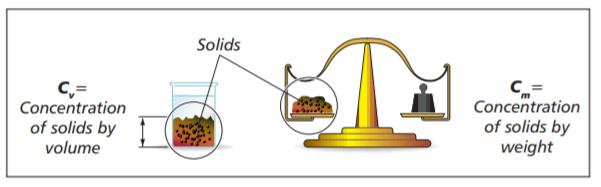Density/Specific Gravity

Solids
The density of the solids is stated as the Specific Gravity. This value, SGs , is determined by dividing he density of the solid by the density of water

Water
The density of water is 1000 kg/m3 . The SG of water is 1,0 at 20°C. The value varies somewhat with temperature.

Slurry
The specific gravity of the slurry can be determined using a nomograph.  Two of the values of SGs, Cv , and Cm, must be known

Particle shape
The shape of the particles is very significant for the behavior of the slurry when pumping and for the wear on the pump and the pipe system. The form factor denotes the deviation of the slurry particles from a perfect sphere.

Slurry characteristics
Slurries can be divided up into settling and non-settling types.

Non-settling slurry
A slurry in which the solids do not settle to the bottom, but remain in suspension for a long time. A non-settling slurry acts in a homogeneous, viscous manner, but the characteristics are non-Newtonian.

Particle size: less than 60-100 µm.
A non-settling slurry can be defined as a homogeneous mixture.

Homogeneous mixture
A mixture of solids and liquid in which the solids are uniformly distributed.

Settling slurry
This type of slurry settles fast during the time relevant to the process, but can be kept in suspension by turbulence. Particle size: greater than 100 µm.
A settling slurry can be defined as a pseudo-homogeneous or heterogeneous mixture and can be completely or partly stratified.

Pseudo-homogeneous mixture
A mixture in which all the particles are in suspension but where the concentration is greater towards the bottom.

Heterogeneous mixture
A mixture of solids and liquid in which the solids are not uniformly distributed and tend to be more concentrated in the bottom of the pipe or containment vessel (compare to settling slurry).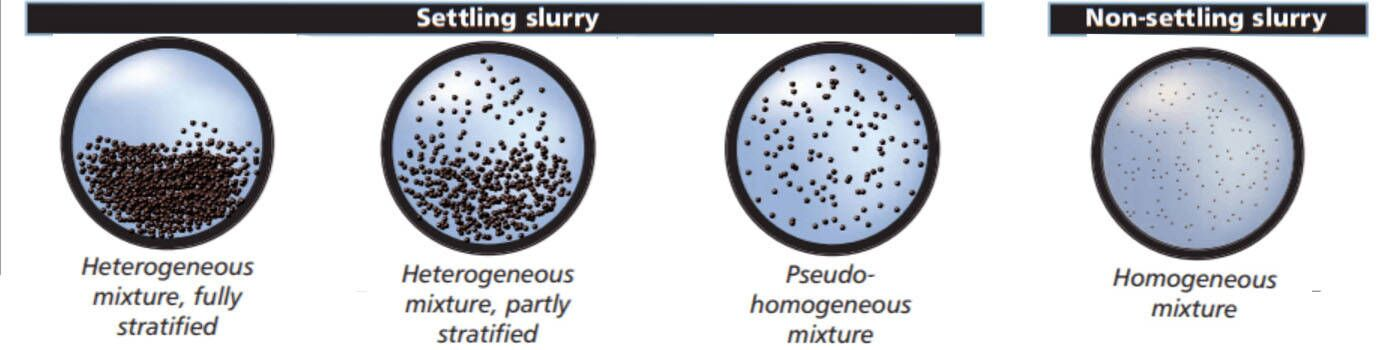Liquid definitions
Except for density, the characteristics of a liquid are decided by its viscosity.

Liquids deform continuously as long as a force is applied to them. They are said to flow. When a flow takes place in a liquid, it is opposed by internal friction arising from the cohesion of the molecules. This internal friction is the property of a liquid called viscosity.

The viscosity of liquids decreases rapidly with increasing temperature.

Newtonian liquids
Newtonian liquids give a shearing stress that is linear and proportional to the velocity gradient, or the shearing rate. Water and most liquids are Newtonian.

Non-Newtonian liquids
Some liquids, such as water based slurries with fine particles, do not obey the simple relationship between shearing stress and shearing rate. They are referred to as

non-Newtonian liquids.
Some non-Newtonian liquids have a unique property of not flowing until a certain minimum shear stress is applied.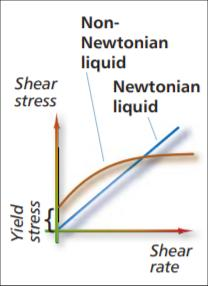Slurry Pump performance
The performance of a centrifugal pump pumping slurry differs from the performance with clean water depending on the amount of solid particles in the slurry.

This difference depends on the characteristics of the slurry (particle size, density, and shape, as described in the previous chapter). The factors that are affected are the power (P), head (H), and efficiency (η). The differences between slurry and water are shown schematically in the curves below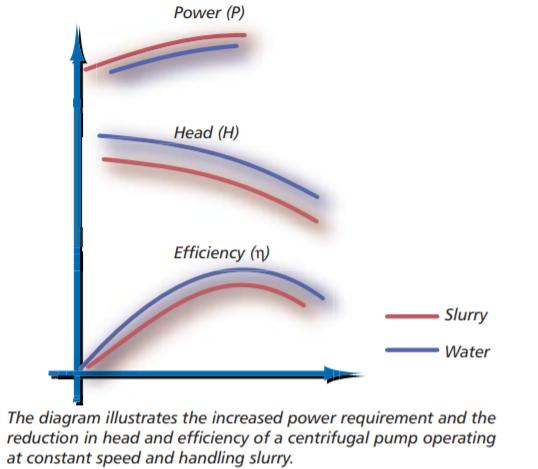System design

Static head is the vertical height difference from the surface of the slurry source to the discharge point.

Friction losses
When the liquid starts to flow through the discharge line and valves, friction will arise. When pumping slurry, friction losses caused by pipe roughness, bends and valves, are different compared to the corresponding losses when pumping water.

This value is used for pump calculations and comprises the static head plus friction losses caused by pipes and valves, converted to meters of water.

Critical velocity
In general, the flow velocity in the pipes must be kept above a certain minimum value. If the flow velocity is too high, friction losses will increase. This may also increase the wear in the pipe system. Flow velocities that are too low will result in sedimentation in the pipes and, thus, increased losses.

This is illustrated in the diagram below, in which the critical velocity (Vc) indicates the optimum velocity where losses are kept to a minimum.

When making calculations for a slurry pump for a certain flow, the desired flow velocity (V) must be compared to the critical velocity (Vc) for the slurry and the pipe system in question. As the figure below shows, the ideal velocity (marked green) is immediately above the critical velocity but with a margin for the extreme cases that can arise.

To determine the critical velocity, the pipe diameter and the particle size (d85) must be known. The value is then corrected with a factor, which depends on the specific gravity of the solids.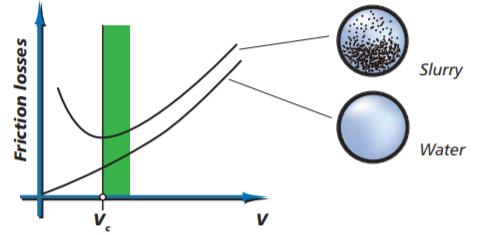Sizing the slurry pump

The diagram above shows, schematically:
1. the pump curve for water
2. the reduced curve for slurry
3. the duty point for slurry, i.e. the point at which the pump system curve and the performance curve intersect.

System curve with correlation for settling slurry effects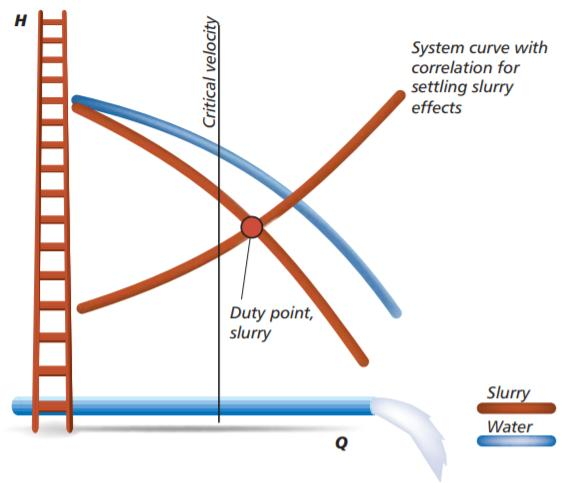Other considerations

Whenever pumps are used, it is important that the pump's inlet pressure exceeds the vapor pressure of the liquid inside the pump. The necessary inlet pressure that is stated for the pump, NPSH req must not be less than the available value in the pump system, NPSH.

The available value depends on the ambient air pressure (height above sea level), the vapor pressure of the liquid, the density of the slurry, and the level in the sump.

Example: Pumping a water-based slurry at a height of 1000 m above sea level. Liquid temperature 40°C, liquid level 2 meters above the pump inlet.

Formula:
NPSHa = air pressure – vapor pressure + level in sump inlet.
NPSHa = 9,2 – 0,4 + 2 = 10,8

None

Post Comment